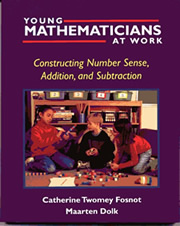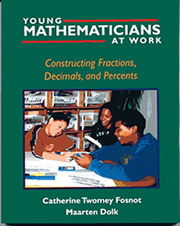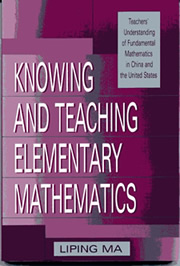Math Books for Professional Educators

Powerful Problem Solving: Activities for Sense Making with Mathematical Practices. 2013: Heinemann, Portsmouth, NH. pages 192
ISBN-13: 078-0325-05090-4This book is an excellent source of information for teachers to learn or review how to facilitate student's mathematical problem solving. The authors apply George Polya's problem solving suggestions, in his book How to Solve It, and the ideas of the NCTM Process Standards: problem solving, representations, connections, communication, and proof and reasoning, to the eight categories of the Common Core Standards for Mathematical Practice. It is an excellent source of detailed - how to information - with specific problem solving activities and suggestions to help teachers faciliate the development of not only problem solving, but students' practices of mathematics.

Mathematicians at Work: Constructing Algebra. 2001: Heinemann, Portsmouth, NH. pages 192
ISBN-13: 978-0-325-02841-5.Excellent information of how mathematical understanding can be facilitated in children with a specific focus on mathematical instruction for constructing mathematical ideas (sign-posts) conceptually for learning algegra.

Mathematicians at Work: Constructing Number Sense, Addition, and Subtraction. 2001: Heinemann, Portsmouth, NH. pages 182
ISBN-13: 0-325-00353-x.Excellent information of how mathematical understanding can be facilitated in children with a specific focus on mathematical instruction for constructing mathematical ideas (sign-posts) conceptually for learning about number value, addition, and subtraction.

Chapter Titles:

1. "Mathematics" or "Mathematizing"?
2. The Landscape of Learning
3. Number Sense on the Horizon
4. Place Value on the Horizon
5. Developing Mathematical Models
6. Addition and Subtraction Facts on the Horizon
7. Algorithms Versus Number Sense
8. Developing Efficient Computation with Minilessons
9. Assessment
10. Teachers as Mathematicians

Mathematicians at Work: Constructing Multiplication and Division. 2001: Heinemann, Portsmouth, NH. pages 159
ISBN-13: 0-325-00354-8.Excellent information of how mathematical understanding can be facilitated in children with a specific focus on mathematical instruction for constructing mathematical ideas (sign-posts) conceptually for learning about multiplication and division.

Chapter Titles

1. Mathematizing  - describes and illustrates what it means to do and learn mathematics
2. The landscape of learning - provides strategies to help teachers turn their classrooms into math workshops that encourage and reflect mathematizing
3. Developing multiplication strategies and big ideas - examines several ways to engage and support children as they construct important strategies and big ideas related to multiplication
4. Connection division to multiplication - takes a close look at the strategies and big ideas related to division
5. Multiplication strategies - defines modeling and provides examples of how learners construct models with a discussion of the importance of context
6. Algorithms versus number sense - discusses what it means to calculate using number sense and whether or not algorithms should still be the goal of computation instruction
7. Developing Efficient Computation with Minilessons
8. Assessment  - describes how to strengthen performance and portfolio assessment
9. Landmark ideas, strategies, models - emphasizes teachers as learners by encouraging them to see themselves as mathematicians.

Mathematicians at Work: Constructing Fractions, Decimals, and Percents. 2002: Heinemann, Portsmouth, NH. pages 159
ISBN-10: 0-325-00353-x.Excellent information of how mathematical understanding can be facilitated in children with a specific focus on mathematical instruction for constructing mathematical ideas (sign-posts) conceptually for learning about fractions, decimals, and percents.

Chapter Titles

1. Mathematizing - describes and illustrates what it means to do and learn mathematics.
2. The landscape of learning - contrasts word problems with true problematic situations which support and enhance investigation and inquiry.
3. Equivalence on the Horizon - provides strategies to help teachers turn their classrooms into math workshops.
4. Developing big ideas and strategies - explores the cultural and historical development of fractions, decimals, and their equivalents and the ways in which children develop similar ideas and strategies.
5. Developing mathematical models  - defines and gives examples of modeling, noting the importance of context.
6. Algorithms vs. number sense  - discusses calculation using number sense and the role of algorithms in computation instruction.
7. Developing efficient computation with minilessons
8. Assessment  - describes how to strengthen performance and portfolio assessment.
9. Landmark ideas, strategies, models - focuses on teachers as learners by encouraging them to see themselves as mathematicians.

Letters to a Young Mathematician. 2006: Joat Enterprises, Portsmouth, NH. pages 203
ISBN-13: 978-0-465-08231-5.A collection of letters written by mathematicians about what it is like being a mathematician. Each can be read alone or as a series. Great reading for anyone interested in math and would be great to read one or two as read alouds in math classes fifth grade and above.

Thinking Mathematically: Integrating Arithmetic and Algebra in Elementary School Outline of Content. 2003: Heinemann, Portsmouth, NH. pages 137
ISBN-10: 0-325-00565-6.Excellent information of how mathematical understanding can be facilitated in elementary students with a specific examples of mathematical instruction of teacher and student interactions for constructing arithmetic and algebra ideas conceptually.

Includes a CD of interviews with children.
Outline and annotations of video clips

Chapter Titles -

1. Developing Mathematical Thinking
2. Equality
3. Developing and Using Relational Thinking
5. Equations with Multiple Variables and Repeated Variables
6. Representing Conjectures Symbolically
7. Justification and Proof
8. Ordering Multiple Operations
9. "If… Then…" Statements: Relations Involving Addition, Subtraction, Multiplication, Division, and Equality

Fostering Algebraic Thinking: A Guide for Teachers Grades 6-10. 1999: Heinemann, Portsmouth, NH. pages 164
ISBN-10: 0-325-00154-5Great ideas for middle school teachers and above to implement as well as important information that al teachers should know about algebraic thinking so preschool and early elementary teachers understand the importance of providing students in the early grades opportunities to explore and play with mathematical ideas concretely so students will begin to connect a variety of external events to mathematical ideas so they will have a solid background of representations to use to construct understanding in algebra in their later grade experiences.

The Roles of Representation in School Mathematics: 2001 Yearbook. 2001: NCTM. pages 282
ISBN-13: 978-0873534956.Want to know more about representation in mathematics K-12. This is a very good source with a collection of articles.

Teacing and Learning Proof Across the Grades: A K-16 Perspective. 2009: Routledge. pages 408
ISBN-13: 978-0415887311.Great ideas about the importance of teaching proof, different types of proofs, and how to implement them to facilitate students learning them K-16.Knowing and Teaching Elementary Mathematics. 2010: , . pages 192
ISBN-13: 978-0805829099.

Knowing and Teaching Elementary Mathematics: Teachers' Understanding of Fundamental Mathematics in China and the United States (Studies in Mathematical Thinking and Learning Series)

Rules or theory - both.

Also a newer version: Knowing and Teaching Elementary Mathematics: Teachers' Understanding of Fundamental Mathematics in China and the United States (Studies in Mathematical Thinking and Learning Series). 2012
ISBN-13: 978-0415873840
232 pages

Children's Mathematics: Cognitively Guided Instrution (CGI). 1999: Heinemann, Portsmouth, NH. pages 110
ISBN-13: 978-0325001371.The Simpsons and their Mathematical Secrets - 2014, Bloomsberry: New York. pages 213 ages 12+.
ISBN-13: 978-1-62040-277-1.Excellent story for anyone that is a fan of the Simpsons, interested in mathematical ideas, trivia nut, Futurama fan, or people that are interested in stories behind the scene stories.

Assorted alphabetical by authors and collections

1. Baratta-Lorton, Mary. (1976). Mathematics Their Way: An Activity Centered Mathematics Program for Early Childhood Education. Menlo Park, CA: Addison-Wesley Publishing Company.
2. Baratta-Lorton, Robert. (1977). Mathematics A Way of Thinking. Menlo Park, CA: Addison-Wesley Publishing Company.
3. Chapin, Suzanne H.; & Johnson, Art. (2000). Math Matters Grades K-6: Understanding the Math You Teach. (2000). Sausalito, CA: Mathematics Solutions Publications.
4. Corwin, Rebecca B., Judith Storeygard, & Sabra L. Price. (1996). Talking Mathematics: Supporting Children's Voices. Portsmouth, NH: Heinemann.
5. Davidson, J. (1983). Using the Cuisenaire Rods. New Rochelle, NY: Cuisenaire Company of America.
6. Grouws, Douglas A. (ed.). (1992). Handbook of Research on Mathematics Teaching and Learning. New York, NY: Macmillan Publishing Company.
7. Hiebert, James; Carpenter, Thomas; Fennema, Elizabeth; Fucson, Karen; Wearne, Diana; Murray, Hanlie; Olivier, Alwyn; & Human, Piet. (1996). Making Sense: Teaching Mathematics with Understanding. Portsmouth, NH: Heinemann.
8. Kamii, C. K. & DeClark, G. (1985). Young Children Reinvent Arithmetic: Implications of Piaget's Theory. New York: Teachers College Press.
9. Kamii, C. K. & Livingston, S. J. (1994). Young Children Continue to Reinvent Arithmetic, 3rd grade: Implications of Piaget's Theory. New York: Teachers College Press.
10. Mills, Heidi; O'Keefe, Timothy; & Whitin, David. (1996). Mathematics in the Making: Authoring Ideas in Primary Classrooms. Portsmouth, NH: Heinemann.
11. Secada, Walter, G editor (1999). Changing the Faces of Mathematics: Perspectives on Latinos. Editors Walter G. Secada, Luis Ortiz_Franco, Norma G. Hernandez, Yolanda De La Cruz. Reston, VI: National Council of Teachers of Mathematics.
12. Smith, Susan Sperry, (2001). Early Childhood Mathematics. Second edition. Needham Heights, MA: Allyn and Bacon.
13. Steen, Lynn Arthur. Editor. (1990). On the Shoulders of Giants: New Approaches to Numeracy. Washington D.C.: National Academy Press.
14. Stenmark, J. K., Thompson, V. & Cossey, R. (1986). Family Math. Berkeley, CA: Lawrence Hall of Science.
15. Theissen, D. & Matthias, M. (1992). The Wonderful World of Mathematics. Reston, VA: National Council of Teachers of mathematics.
16. Trafton, P. & Theissen, D. (1999). Learning through Problems: Computational Strategies: A Resource for Teachers. Portsmouth, NH: Heinemann.

NCTM sources

• Courtney Koestler, Mathew D. Felton, Kristen N. Bieda, & Samuel Otten. (2013). Connecting the NCTM Process Standards & the CCSSM Practices. Reston, VA: National Council of Teachers of mathematics.
• (2000). Principles and Standards for School Mathematics. Reston, VA: National Council of Teachers of Mathematics.
• (2001). Navigation Series. Navigating through ... all content topics and grades K-12. Reston, VA: The National Council of Teachers of Mathematics, INC.
• (1998). 1998 Yearbook The Teaching and Learning of Algorithms in School Mathematics. Reston, VA: The National Council of Teachers of Mathematics, INC.
• (1999). 1999 Yearbook Developing Mathematical Reasoning in Grades K-12. Reston, VA: The National Council of Teachers of Mathematics, INC.
• (2000). 2000 Yearbook Learning Mathematics for a New Century. Reston, VA: The National Council of Teachers of Mathematics, INC.
• (2001). 2001 Yearbook The Roles of Representation in School Mathematics. Reston, VA: The National Council of Teachers of Mathematics, INC.